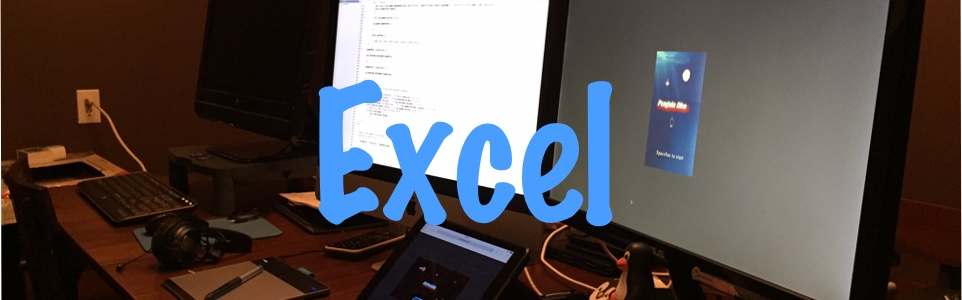In this section of the Web Site you will find some Excel examples that I have put together over the years. Ranging from simple Excel formula based files to more complex Excel files using VBA for Excel and a XLL add-in example. Please feel free to download and use any of the examples. I have been moving away from Excel over the last few years, because even though I know VBA not many people do and maintaining a spreadsheet became a nightmare.

#### Excel using Formula's only

Conditional Format - In this file you will find a simple example of how to highlight a row that is past a certain due date.

Sum between dates - In this example you will find a simple vlook-up that sum a range of numbers between dates.

Various Examples of Sum If - In this example you will find various examples of Excels Sum If function.

#### Excel using VBA

VBA Examples of adding up a range of numbers by color

Example of a simple VBA form - Calculate Discount shows how to use a VBA form and link it back into Excel

Examples of custom functions in Excel and how to link them back into a Excel tab.

More Excel Examples